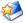# [原创]中间运力型火箭是21世纪列强们的新一代帝国名片

1，独立进行载人登月，要单独发射登月舱，LTO运力达20吨是关键的火箭运力节点。

2，要独立发射联盟飞船与神舟飞船的NRHO版本，LTO运力达12吨是关键的火箭运力节点。

3，实施载人登月工程，无论是对于美俄还是中国，2028年都是关键的时间节点。

打赏
收藏文本
0
0
2019/3/5 8:47:23

## 网友回复

左箭头-小图标

[size=10.5]经济贫穷的俄罗斯注定难以研制出[size=10.5]LTO[size=10.5]运力达[size=10.5]20[size=10.5]吨以上的重型火箭。[size=10.5]
[size=10.5]
[size=10.5]目前[size=10.5]921[size=10.5]载人火箭进展神速，长征五号火箭末来己经不可能用于执行载人飞船的发射任务。[size=10.5]
[size=10.5]
[size=10.5]但未来中国是研制重达[size=10.5]10[size=10.5]至[size=10.5]12[size=10.5]吨级的[size=10.5]NRHO[size=10.5]版本神舟载人飞船，还是研制重达[size=10.5]23[size=10.5]吨的全新月球载人飞船，现在则远远未能明朗化。[size=10.5]
[size=10.5]
[size=10.5]研制[size=10.5]NRHO[size=10.5]版本的神舟载人飞船技术风险低、投资需求小，而且未来完全回收复用版本的[size=10.5]921[size=10.5]载人火箭都能够执行发射任务，其的运营经济性非常之好。缺点则是技术老旧，载人少（最多只能运载[size=10.5]3[size=10.5]名宇航员到达[size=10.5]NRHO[size=10.5]月球空间站）。[size=10.5]
[size=10.5]
[size=10.5]而全新的[size=10.5]23[size=10.5]吨级[size=10.5]2[size=10.5]舱版月球载人飞船则刚好相反，研制技术风险高、投资成本需求大，未来完全回收复用版本的[size=10.5]921[size=10.5]运载火箭无法进行发射，其的运营经济性则要差很多。但优点是能够推进技术创新，并且载人的数量也多。[size=10.5]
[size=10.5]
[size=10.5]因此，最后中国政府是决定上马研制[size=10.5]NRHO[size=10.5]版本的神舟载人飞船还是[size=10.5]23[size=10.5]吨级的全新月球载人飞船，内部肯定还存在一番争吵不休的“大论战”的。[size=10.5]
[size=10.5]
[size=10.5]当然，从个人的角度而言，如果政府投资的钱足够多，高凉陈君也极力支持上马研制重达[size=10.5]23[size=10.5]吨的大飞船。毕竟无论如何，住豪宅都要比住蜗居要舒服得多。[size=10.5]
[size=10.5]
[size=10.5]但俄罗斯则不然，俄罗斯人未来[size=10.5]99%[size=10.5]都只能研制出一款[size=10.5]LTO[size=10.5]运力达[size=10.5]10[size=10.5]吨左右的[size=10.5]NRHO[size=10.5]版联盟载人飞船来应付一下此轮载人登月竞赛就了事。核心原因就是俄罗斯人没钱研制出一款[size=10.5]LTO[size=10.5]运力高达[size=10.5]20[size=10.5]吨以上的重型火箭来。[size=10.5]
[size=10.5]
[size=10.5]毕竟研制[size=10.5]LTO[size=10.5]运力只有[size=10.5]10[size=10.5]吨左右的重型火箭与研制[size=10.5]LTO[size=10.5]运力高达[size=10.5]20[size=10.5]吨以上的重型火箭相比，投资需求的差距还是非常巨大的。俄罗斯人现在一没有钱研制直径达[size=10.5]8[size=10.5]米以上的新主芯级火箭，二没有钱研制氢氧上面级火箭。因此仅仅使用常温上面级，要“堆”出[size=10.5]LTO[size=10.5]运力[size=10.5]10[size=10.5]吨左右的重型火箭还可以。但要指望使用常温上面级，却还要“堆”出[size=10.5]LTO[size=10.5]运力高达[size=10.5]20[size=10.5]吨以上的中间运力型火箭，可就真正“难比登天”了。[size=10.5]

[size=10.5]以目前俄罗斯人现成的火箭工业生产能力，基于质子火箭与安加拉[size=10.5]5[size=10.5]火箭的硬件基础，要拼凑出一款[size=10.5]LTO[size=10.5]运力达[size=10.5]10[size=10.5]吨左右的重型火箭完全可行。但要研制出[size=10.5]LTO[size=10.5]运力高达[size=10.5]20[size=10.5]吨以上的重型火箭可就绝对没有那么简单，起码一款能够“堪用”的低温氢氧发动机上面级都是要有的。问题是今天俄罗斯的氢氧发动机生产能力还存在不存在？！而要重建俄罗斯氢氧发动机与氢氧箭体的生产能力，未来没有三、四十亿美元的投入，想都不要想。[size=10.5]
[size=10.5]
[size=10.5]因此一分钱难倒英雄汉，现在的俄罗斯人有想法也注定没有办法。[size=10.5]
[size=10.5]
[size=10.5]陈天（高凉陈君）[size=10.5]
[size=10.5]

2019/3/5

2019/3/5 17:04:19
左箭头-小图标

[size=10.5]总结[size=10.5]
[size=10.5]
[size=10.5]921[size=10.5]载人火箭的研制现在明显是快马加鞭、日夜兼程。而且随着[size=10.5]2028[size=10.5]年关键时间节点的逐年迫近，其具体的研制时间进度表，“上头”只会越抓越严格。但未来只要中国[size=10.5]LTO[size=10.5]运力高达[size=10.5]25[size=10.5]吨的中间运力型火箭研制成功，就真正搬开了阻碍中国人登上月球的最重大的一座大山。[size=10.5]
[size=10.5]
[size=10.5]与之相反，剩下的俄、日、欧三方，随着时间的推移就只能够越来越纠结了。[size=10.5]
[size=10.5]
[size=10.5]俄罗斯人现在有野心又有技术，但却缺乏钱。而欧洲人与日本人有技术有钱，但却缺乏野心。因此随着美国的猎鹰[size=10.5]9H[size=10.5]、[size=10.5]SLS[size=10.5]与中国的[size=10.5]921[size=10.5]重型火箭的次递投入使用运营，欧、日、俄三方政府再不进行“国家级的总动员（参考阿波罗时代美国与苏联政府的状态）”，后果注定只会越来越被动。[size=10.5]
[size=10.5]
[size=10.5]还是那句话，列强帝国间的载人登月竞赛不是你想回避就回避得了的，不想做[size=10.5]21[size=10.5]世纪的大清政府，就必须直面挑战、迎难而上、奋勇前进。[size=10.5]
[size=10.5]
[size=10.5]陈天（高凉陈君）[size=10.5]
[size=10.5]

2019/3/5

2019/3/5 15:07:47
左箭头-小图标

[size=10.5]讲困难是没有用的。当年大英帝国上马研制英王乔治五世、日本研制大和、法国研制黎塞留、意大利研制维内托、德国研制俾斯麦与美国研制依阿华战列舰也一样困难重重。但最后经过种种艰苦努力，也都各自研制成功了。[size=10.5]
[size=10.5]
[size=10.5]至于说到研制[size=10.5]LTO[size=10.5]运力达到[size=10.5]10[size=10.5]至[size=10.5]15[size=10.5]吨区间的中间运力型火箭，末来在军事上有没有用，这显然是无须置疑的问题。只要研制出来了，自然迟早就会有国家会使用到军事方面上去的（如发射军事空间站）。[size=10.5]
[size=10.5]
[size=10.5]多谢上面的朋友提到的巴兹太空计划的信息。我从来不知道还有这种的一款游戏（我实际上也从来不接触网络游戏）。百度百科关于巴兹太空计划游戏内容的简介里面，就有载人飞船地球交汇计划与双子星座载人飞船直升（直接奔月）计划的介绍。[size=10.5]
[size=10.5]
[size=10.5]因此如果未来中国研制了[size=10.5]NRHO[size=10.5]版本的神舟飞船（[size=10.5]2[size=10.5]人版），俄罗斯研制了[size=10.5]NRHO[size=10.5]版本的联盟飞船（[size=10.5]2[size=10.5]人版），实际上阿波罗时代麦道公司提出的双子星座飞船直接奔月计划就能够立即成功复活。这也非常适合没有办法、没有能力研制巨型火箭的国家拿来借鉴参考。[size=10.5]
[size=10.5]
[size=10.5]NRHO[size=10.5]版本的神舟飞船与联盟飞船，本质上就是阿波罗时代原苏联联盟[size=10.5]7KL1[size=10.5]与联盟[size=10.5]7KL3[size=10.5]月球载人飞船的现代技术复活升级版。总重量能够控制于[size=10.5]10[size=10.5]（[size=10.5]2[size=10.5]人版）至[size=10.5]12[size=10.5]吨（[size=10.5]3[size=10.5]人版）的区间。只要中国与俄罗斯政府肯进行投资，就绝对能够在短时间（[size=10.5]5[size=10.5]年）内研制出来。[size=10.5]

[size=10.5]也都是典型的“短、平、快”研制项目，能够被方方面面的势力所接受。现在就看中俄政府的决策态度了。[size=10.5]

[size=10.5]第三[size=10.5]

NRHO[size=10.5]版本的神舟飞船与联盟飞船研制技术简单、投资需求适中，中俄两国要研制与之配套的[size=10.5]LTO[size=10.5]运力达[size=10.5]10[size=10.5]至[size=10.5]12[size=10.5]吨区间的重型火箭也非常容易。[size=10.5]

[size=10.5]
[size=10.5]因此，高凉陈君认为未来[size=10.5]10[size=10.5]年时间内中俄两国政府通过研制[size=10.5]NRHO[size=10.5]版本的神舟飞船与联盟飞船，就能够将各自的月球空间站的载人运输基本框架迅速搭建出来（即解决掉各自[size=10.5]NRHO[size=10.5]月球空间站宇航员运载能力的空白问题）。之后，如果投资资金充足，中国政府可以接着同时上马[size=10.5]NRHO[size=10.5]月球空间站（[size=10.5]25[size=10.5]吨级）与载人登月舱两大后继工程。再通过中国小型[size=10.5]NRHO[size=10.5]月球空间站“集合与中转”，就可以迅速实现中国载人登陆月球的千年梦想。[size=10.5]
[size=10.5]
[size=10.5]但如果后继不想投资太多的钱，中国政府也可以仅仅上马迷你版[size=10.5]NRHO[size=10.5]月球空间站研制项目（[size=10.5]15[size=10.5]吨左右，即地球版的天宫太空实验室（[size=10.5]8[size=10.5]吨）再配上一个具备[size=10.5]2[size=10.5]个对接口的节点舱（[size=10.5]7[size=10.5]吨）就了事），先为中国宇航员在月球[size=10.5]NRHO[size=10.5]轨道找到一个落脚点再说。[size=10.5]
[size=10.5]
[size=10.5]总而言之，未来[size=10.5]10[size=10.5]年时间内研制[size=10.5]NRHO[size=10.5]版本的神舟飞船与联盟飞船对于中俄两国而言都是典型的“进可攻与退可守”的基础性重大空间项目。[size=10.5]
[size=10.5]
[size=10.5]反正欧洲与日本现在已经完全放弃独立自主研制月球载人飞船的所有梦想了。因此末来[size=10.5]10[size=10.5]年时间内无论中国还是俄罗斯，只要能够独立研制出自己的[size=10.5]NRHO[size=10.5]版本的月球载人飞船，就绝对是“向上一小步、也是新高峰”的里程碑式伟大历史成就。[size=10.5]
[size=10.5]
[size=10.5]陈天（高凉陈君）[size=10.5]
[size=10.5]

2019/3/5

2019/3/5 8:50:18

总页数11页 [共有4条记录] 分页：

1
对[原创]中间运力型火箭是21世纪列强们的新一代帝国名片回复